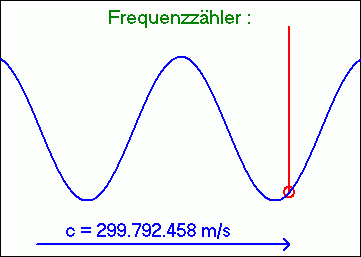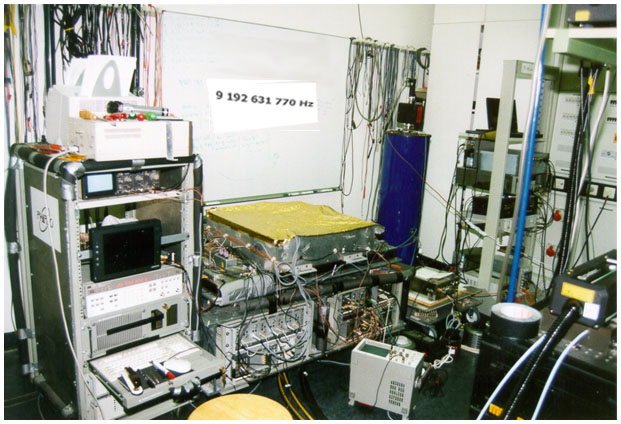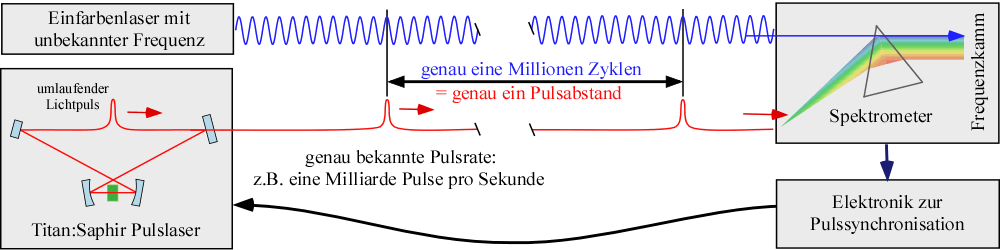# Which wave has the highest frequency

Let there be light
 Electromagnetic waves are characterized by their frequency f and their wavelength l characterized. The frequency can be determined from the wavelength l about the relationship f = c / l to calculate. It is c the speed of light of 299,792,458 meters per second. If the wavelength of the electromagnetic radiation is between 400 and 800 nanometers (1 nanometer = 1 millionth of a millimeter), it is visible light. We perceive the different wavelengths as different colors. The longest visible wavelengths appear red and the shortest appear blue. Light with a larger or smaller wavelength is accordingly referred to as infrared or ultraviolet radiation. The frequencies of visible light are far too great to be counted electronically..

Highest frequencies

 The highest electronic frequencies that are used are, for example, for satellite television or in a modern PC (around 1-10 gigahertz). Green light, on the other hand, with a wavelength of 500 nanometers has a frequency of 600,000 gigahertz (1 gigahertz = 1 billion oscillations per second). For this reason, in the past, the wavelength of light and not its optical frequencies were always measured in spectroscopic applications. We owe most of our knowledge about the physics of atoms to spectroscopy - the determination of the wavelengths of the light emitted by atoms. In order to test the validity of the theories developed from it (for example quantum mechanics), one has to measure very precisely, because these theories also allow very precise calculations. In fact, in the case of quantum mechanics, no significant deviation from the experiment has yet been found, although the measurement accuracy has increased dramatically in the last few decades.Highest accuracy

 Today you can build clocks that would go wrong by just 5 minutes since the beginning of the universe about 10 billion years ago. All other physical quantities except time can only be measured with much less precision. So in order to achieve maximum accuracy one has to measure a time somehow. This is achieved with a frequency measurement, i.e. the determination of the number of oscillations per second. If you are not mistaken when counting and have a very large number of oscillations, a frequency measurement is just as accurate as the clock that tells you when the second is over. For example, in our laboratory, a transition frequency in the hydrogen atom, which is of central importance for basic research, was determined to be 2,466,061,413,187,103 ± 46 oscillations per second.The most accurate clock in the world from the LPTF / Paris in ours Garching laboratory.

The optical frequency comb

To create an optical frequency comb, we use a titanium: sapphire pulse laser, in which a very short light pulse circulates via deflecting mirrors (bottom left). The energy losses due to less than perfect reflection and due to the coupling out of part of the pulse intensity through a partially transparent mirror are compensated for by a built-in optical amplifier (the titanium: sapphire crystal in the figure in green) so that the same pulse can circulate in the mirror arrangement for days . A copy of the light pulse is obtained at the output of the laser after each cycle (once per nanosecond). With the help of a trick we invented, the pulse rate can be set in such a way that exactly one pulse falls on exactly one million cycles of a very fast oscillating single-color laser. This is done with the help of a spectrometer that analyzes the light from the pulsed laser. When viewed very closely, the spectrum of the pulse laser has similarities with a comb, hence the name frequency comb. There are about a million "prongs" in this comb. By superimposing the single-color laser with the frequency comb on a photodetector, electrical signals can be generated that can be used for pulse synchronization. To determine the frequency of the monochrome laser, one only needs to determine the easily measurable pulse rate (about 1 GHz) and then multiply this by a million. In this sense, the frequency comb is a kind of frequency divider that reduces the frequency of the single-color laser, which is far too high, to the electronically countable frequency of the pulse laser.The next step: the optical clock
A clock, whether it is a sundial, hourglass, pendulum clock, quartz clock or cesium atomic clock, always consists of two components, an oscillator that vibrates as evenly as possible and a counter that counts these vibrations and, after a certain number, moves the second hand by one unit, for example . While with clocks with very slow oscillators, for example with the sundial with one oscillation per day, people can count and move the "day hand" further by one, with pendulum clocks you need a counter in order to have a practically usable clock. With a quartz clock or a cesium atomic clock, in which the cesium atoms vibrate exactly 9,192,631,770 times per second (this is how the second is defined), you need an electronic counter. When comparing the different clocks it is noticeable that the faster the pendulum swings, the more precise they are. That is why you could build a much more accurate clock with an "optical" pendulum. As an optical pendulum, you become an atom that emits a precisely defined optical wave, i.e. light. This pendulum has not been the greatest technical challenge so far, but the clockwork that can count such fast oscillations. With the frequency comb, such a counter can now be implemented in the simplest way.

And why is it all?
Accurate atomic clocks are needed to synchronize data networks. For example, when sending a fax, an atomic clock may be used. Another application from everyday life is satellite navigation in the car or on the plane. With the more precise optical clocks, however, new applications may arise, such as the detection of ore deposits through their gravitation and the associated tiny change in the course of time (keyword: time dilation, general relativity). However, we originally developed this technology at our institute for basic research. We have thus checked the precise predictions of quantum mechanics based on the hydrogen atom to a total of 14 decimal places. Quantum mechanics is the most accurate, but also the strangest theory that physicists have ever had. Because this theory contradicts common sense even more than the theory of relativity, attempts have been made to involve it in contradictions with nature for almost 100 years. So far, however, unsuccessful. Our experiments show that quantum mechanics is also right when it comes to the fourteenth decimal place. As a small consolation it remains that this strange theory can in the meantime "explain" almost all natural phenomena correctly, but persistently remains silent about others, such as gravity.

Thomas Udem October 4th, 2005